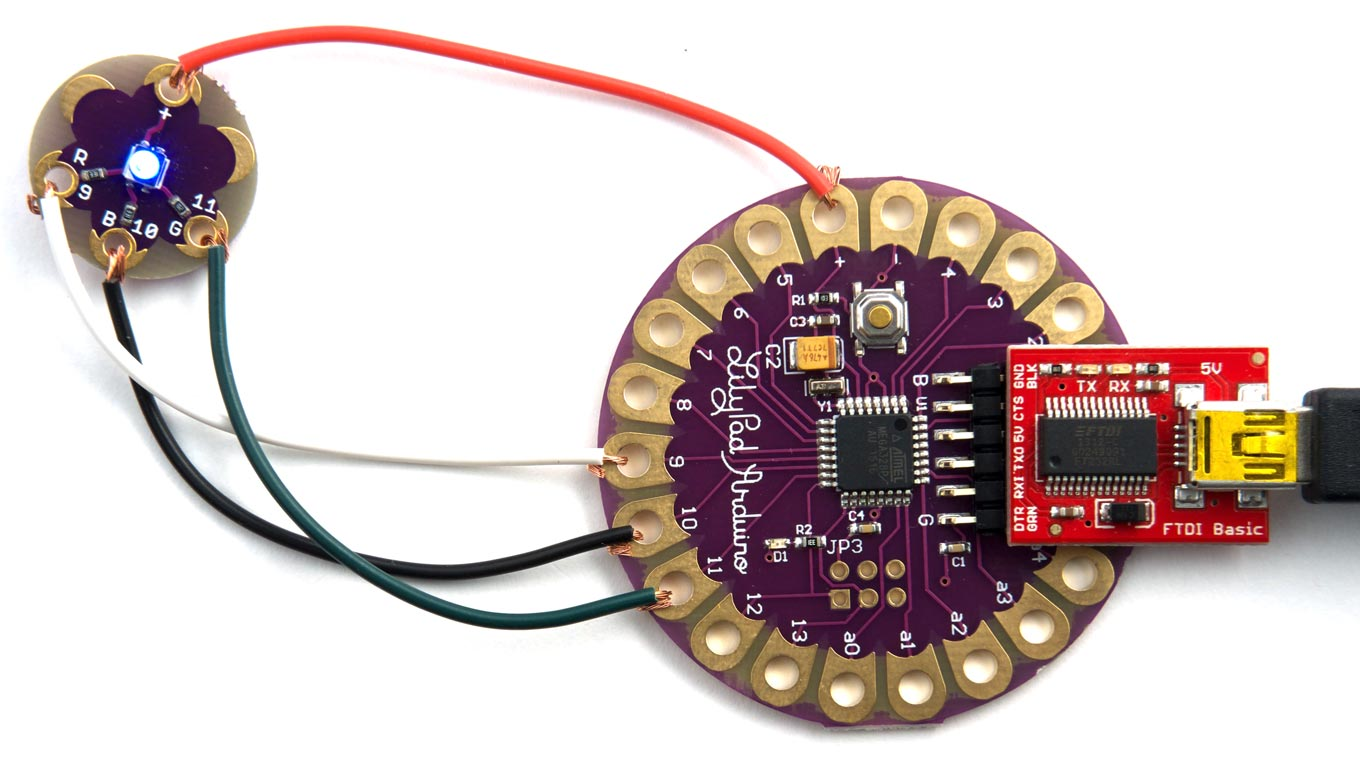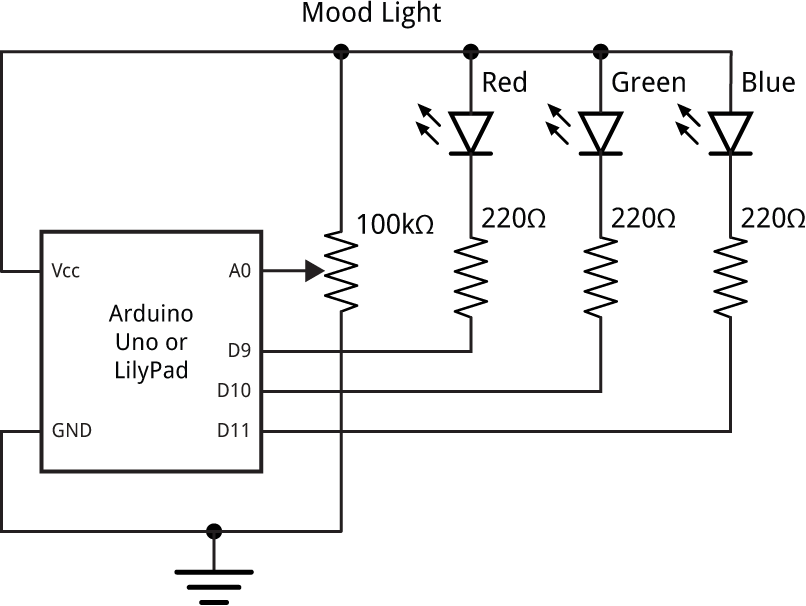## Mood light

This simple example gradually changes the colour of an RGB LED, smoothly varying it between red, magenta, blue, cyan, green, yellow, orange, and back to red. It will run on an Arduino Uno, Arduino Mega 2560, or any equivalent board such as the LilyPad:Mood light programmed in uLisp running on an Arduino LilyPad.

### The circuit

Here's the circuit:The circuit of the mood light; the potentiometer is optional.

### The uLisp program

The program will go through 768 steps, ramping each colour up to maximum and then back down to zero in turn.

First we define three functions, red, grn, and blu, to give the intensity of each light for a value from 0 to 767. The LilyPad RGB LED is wired so that 0 gives maximum brightness and 255 turns the LED off, so the definition of red is:

```(defun red (x)
(let ((y (mod x 768)))
(min
(if (> y 255) (- y 255) (- 255 y))
255)))```

See below for an alternative definition if your LEDs are wired the other way round.

The functions grn and blu simply call red with a displacement of 256 and 512 steps respectively:

```(defun grn (x) (red (+ x 256)))
(defun blu (x) (red (+ x 512)))```

This routine rgb sets the red, green, and blue LEDs on pins 9, 10, and 11 to the appropriate values:

```(defun rgb (v)
(mapc
analogwrite
'(9 10 11)
(list (red v) (grn v) (blu v))))```

Finally, run cycles the LEDs through the different colours in a loop:

```(defun run ()
(let ((x 0))
(loop
(rgb x)
(setq x (mod (1+ x) 768))
(delay 10))))```

For a more subtle effect increase the delay to 100.

To run the mood light enter:

`> (run)`

To exit from the loop type a "~" and press return.

Alternatively if you want to be able to adjust the colour of the light yourself, add a potentiometer as shown in the above circuit diagram, and run the following pot function:

`(defun pot () (loop (rgb (analogread 0))))`

### LEDs wired to ground

If your LEDs are wired to ground, so 255 gives maximum brightness and 0 turns them off, change red to:

```(defun red (x)
(let ((y (mod x 768)))
(max
(if (> y 255) (- 511 y) y)
0)))```

Previous: Tweetmaze